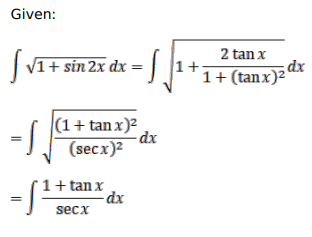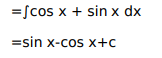# Mark against the correct answer in each of the following:

Question:

Mark $(\sqrt{ })$ against the correct answer in each of the following:

$\int \sqrt{1+\sin 2 x} d x=?$

A. $\sin x+\cos x+C$

B. $-\sin x+\cos x+C$

C. $\sin x-\cos x+C$

D. $-\sin x-\cos x+C$

Solution: